# Ch 25 Electric Potential Energy Warmup 05 Electric

• Slides: 30Ch. 25 Electric Potential Energy Warmup 05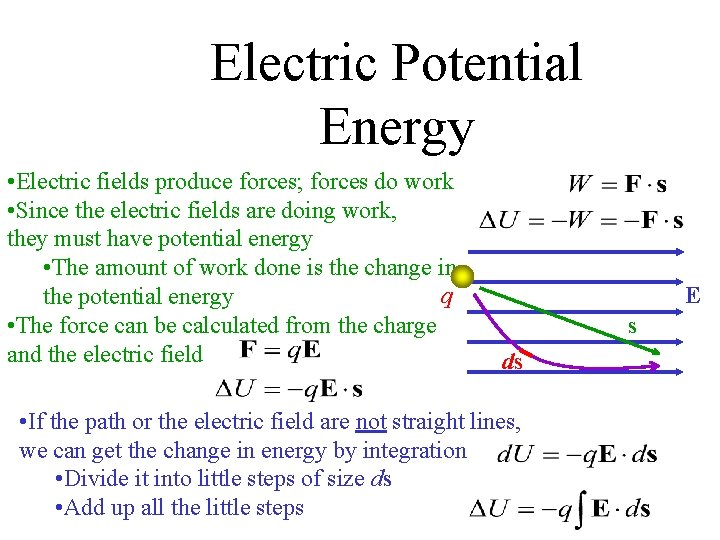Electric Potential Energy • Electric fields produce forces; forces do work • Since the electric fields are doing work, they must have potential energy • The amount of work done is the change in q the potential energy • The force can be calculated from the charge and the electric field E s ds • If the path or the electric field are not straight lines, we can get the change in energy by integration • Divide it into little steps of size ds • Add up all the little steps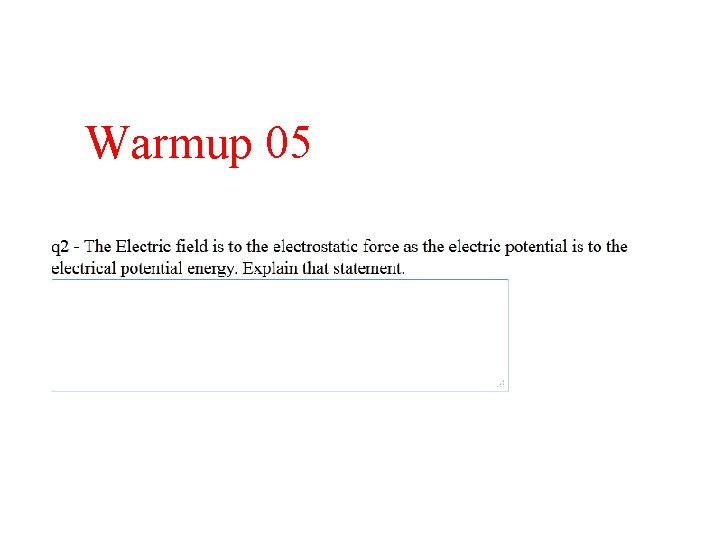Warmup 05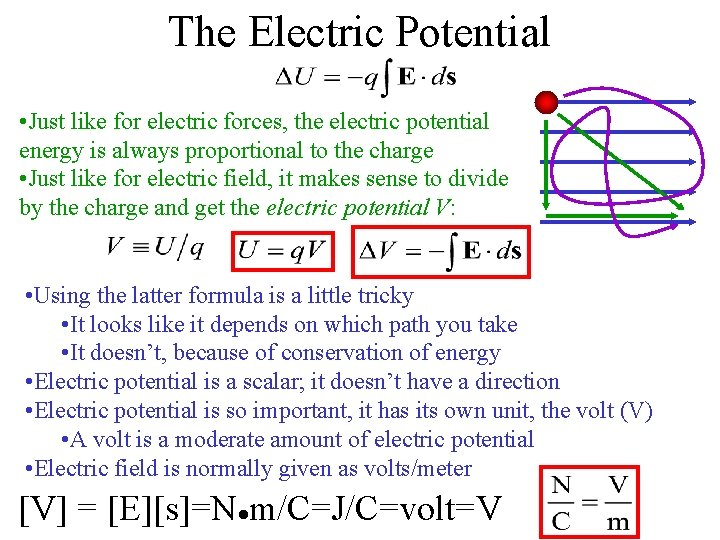The Electric Potential • Just like for electric forces, the electric potential energy is always proportional to the charge • Just like for electric field, it makes sense to divide by the charge and get the electric potential V: • Using the latter formula is a little tricky • It looks like it depends on which path you take • It doesn’t, because of conservation of energy • Electric potential is a scalar; it doesn’t have a direction • Electric potential is so important, it has its own unit, the volt (V) • A volt is a moderate amount of electric potential • Electric field is normally given as volts/meter [V] = [E][s]=N●m/C=J/C=volt=V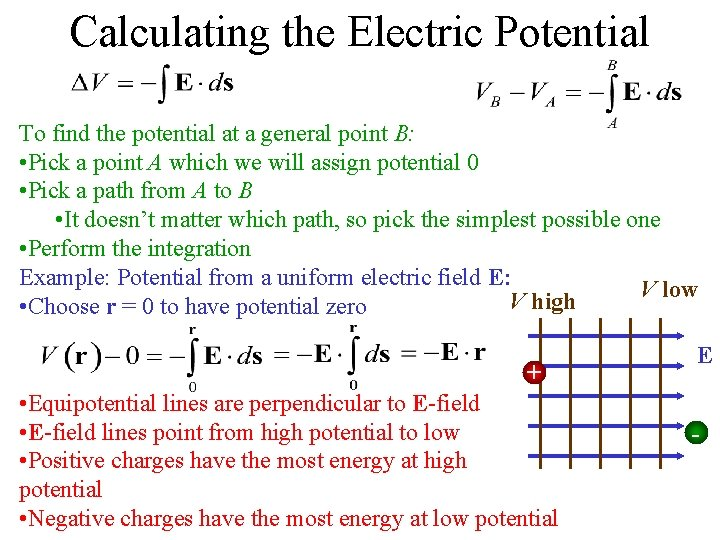Calculating the Electric Potential To find the potential at a general point B: • Pick a point A which we will assign potential 0 • Pick a path from A to B • It doesn’t matter which path, so pick the simplest possible one • Perform the integration Example: Potential from a uniform electric field E: V low V high • Choose r = 0 to have potential zero + • Equipotential lines are perpendicular to E-field • E-field lines point from high potential to low • Positive charges have the most energy at high potential • Negative charges have the most energy at low potential E -JIT Quick Quiz 25. 1 Ans (i) b (ii) a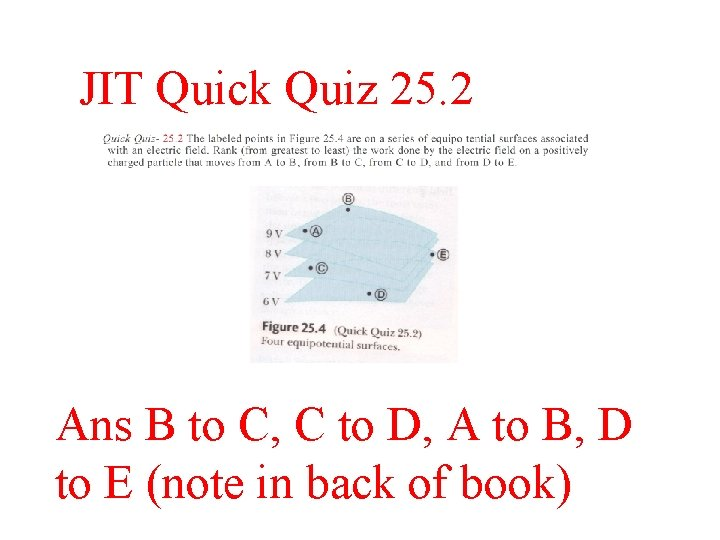JIT Quick Quiz 25. 2 Ans B to C, C to D, A to B, D to E (note in back of book)Solve on Board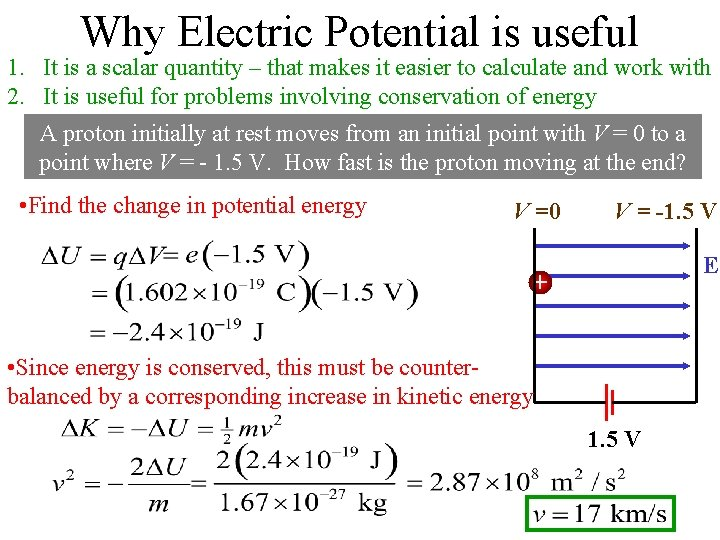Why Electric Potential is useful 1. It is a scalar quantity – that makes it easier to calculate and work with 2. It is useful for problems involving conservation of energy A proton initially at rest moves from an initial point with V = 0 to a point where V = - 1. 5 V. How fast is the proton moving at the end? • Find the change in potential energy V =0 V = -1. 5 V E + • Since energy is conserved, this must be counterbalanced by a corresponding increase in kinetic energy 1. 5 VWarmup 05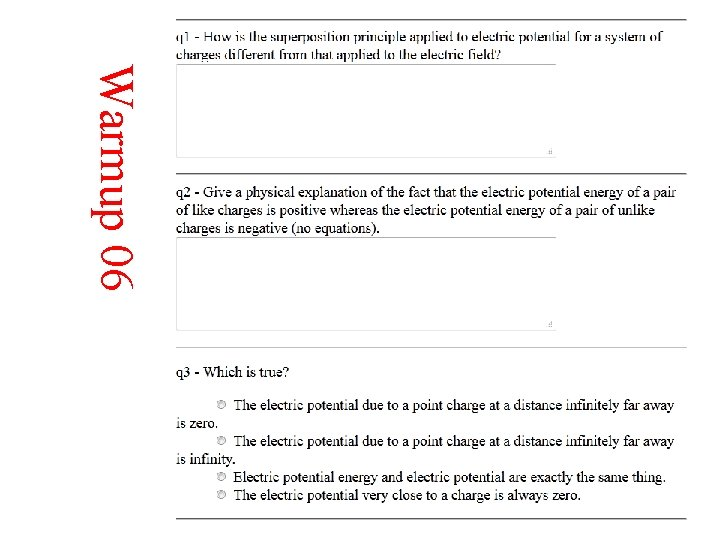Warmup 06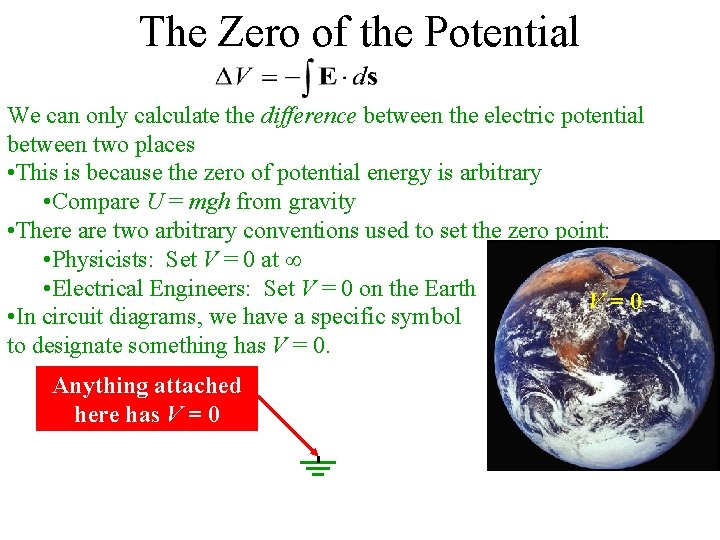The Zero of the Potential We can only calculate the difference between the electric potential between two places • This is because the zero of potential energy is arbitrary • Compare U = mgh from gravity • There are two arbitrary conventions used to set the zero point: • Physicists: Set V = 0 at • Electrical Engineers: Set V = 0 on the Earth V=0 • In circuit diagrams, we have a specific symbol to designate something has V = 0. Anything attached here has V = 0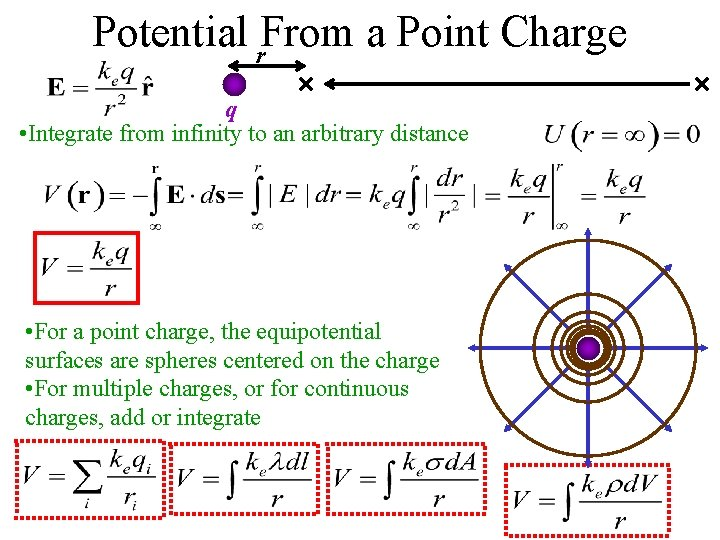Potential r. From a Point Charge q • Integrate from infinity to an arbitrary distance • For a point charge, the equipotential surfaces are spheres centered on the charge • For multiple charges, or for continuous charges, add or integrate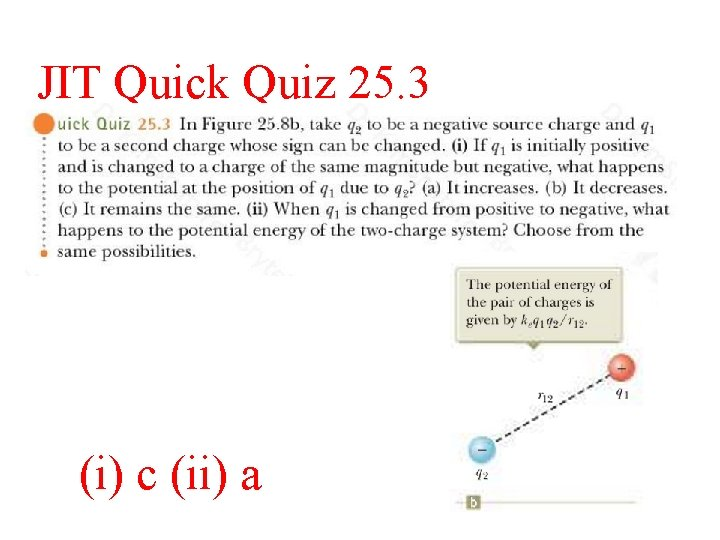JIT Quick Quiz 25. 3 (i) c (ii) a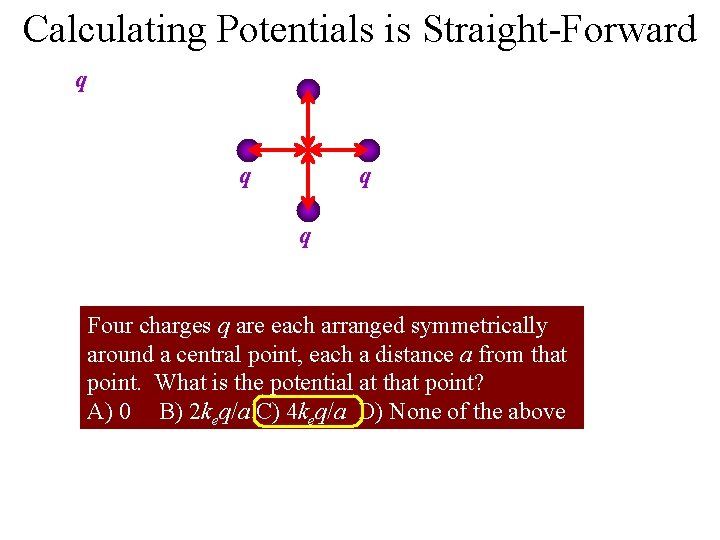Calculating Potentials is Straight-Forward q q Four charges q are each arranged symmetrically around a central point, each a distance a from that point. What is the potential at that point? A) 0 B) 2 keq/a C) 4 keq/a D) None of the aboveAns B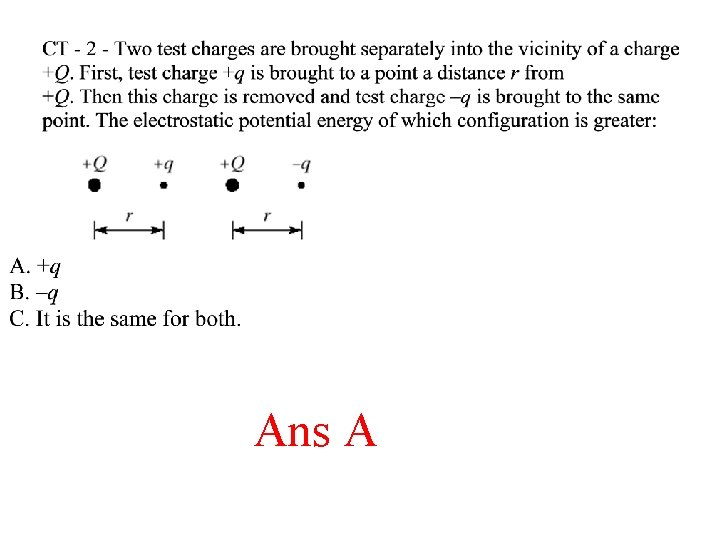Ans A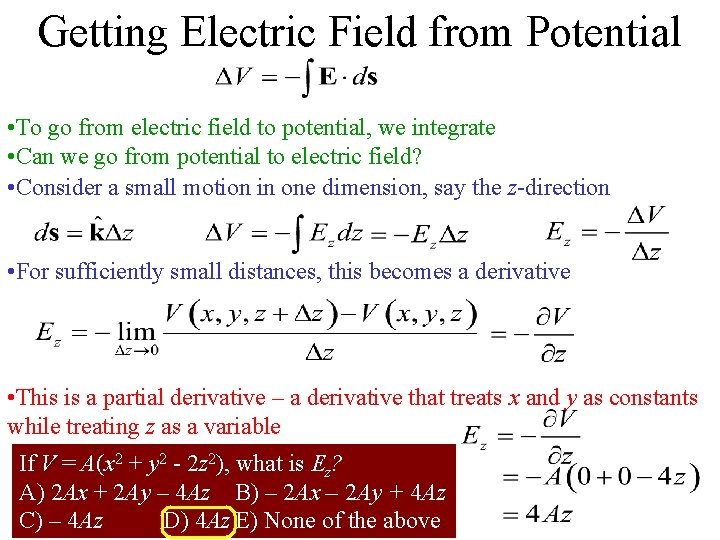Getting Electric Field from Potential • To go from electric field to potential, we integrate • Can we go from potential to electric field? • Consider a small motion in one dimension, say the z-direction • For sufficiently small distances, this becomes a derivative • This is a partial derivative – a derivative that treats x and y as constants while treating z as a variable If V = A(x 2 + y 2 - 2 z 2), what is Ez? A) 2 Ax + 2 Ay – 4 Az B) – 2 Ax – 2 Ay + 4 Az C) – 4 Az D) 4 Az E) None of the above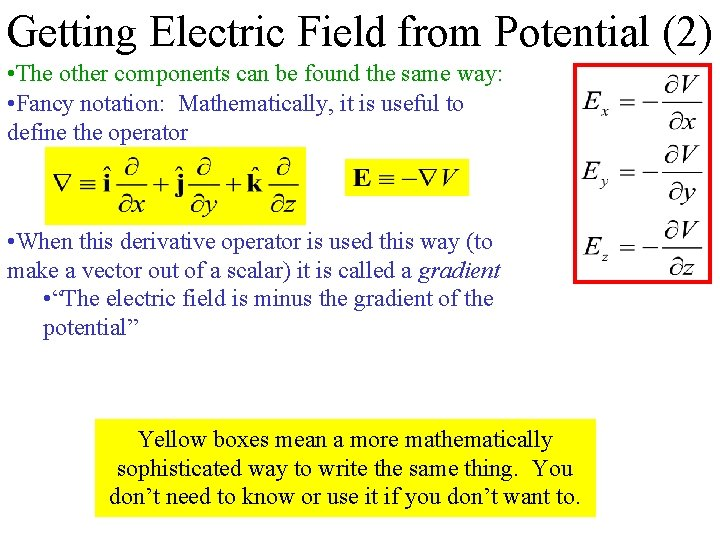Getting Electric Field from Potential (2) • The other components can be found the same way: • Fancy notation: Mathematically, it is useful to define the operator • When this derivative operator is used this way (to make a vector out of a scalar) it is called a gradient • “The electric field is minus the gradient of the potential” Yellow boxes mean a more mathematically sophisticated way to write the same thing. You don’t need to know or use it if you don’t want to.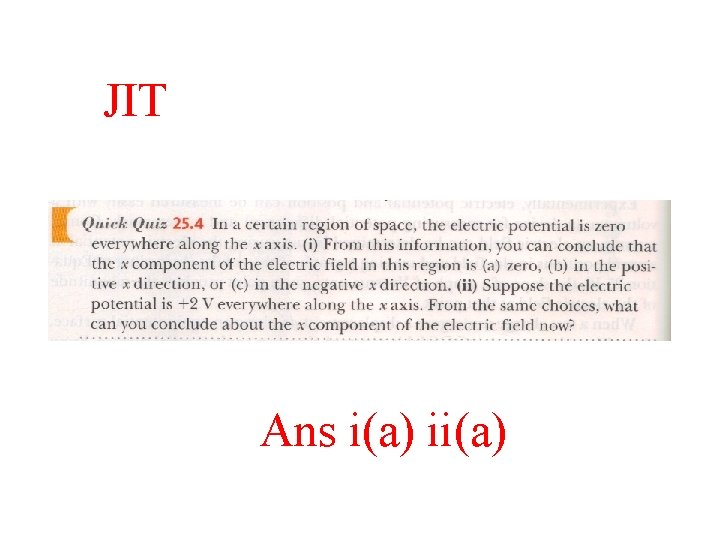JIT Ans i(a) ii(a)Solve on BoardEquipotential Lines Are Like Topographical Maps • Regions of high potential are like “mountains” • For positive charges, they have a lot of energy there • Regions of low potential are like “valleys” • For positive charges, they have minimum energy there • Electric fields point down the slope • Closely spaced equipotential lines means big electric fieldUnderstanding Equipotential Lines In the graph below, what type of charge is at X, and what at Y? A) Positive, both places B) Positive at X, negative at Y C) Negative at X, positive at Y D) Negative, both places • Positive charges don’t want to climb the high -1 potentials in k. V +1 0 mountain at Y -2 +2 • Must be positive charge repelling -3 them! X-4 Y +4 +3 • Positive charges want to flow into low valley at X • Must be negative charge attracting them! • Electric fields are perpendicular to equipotential surfaces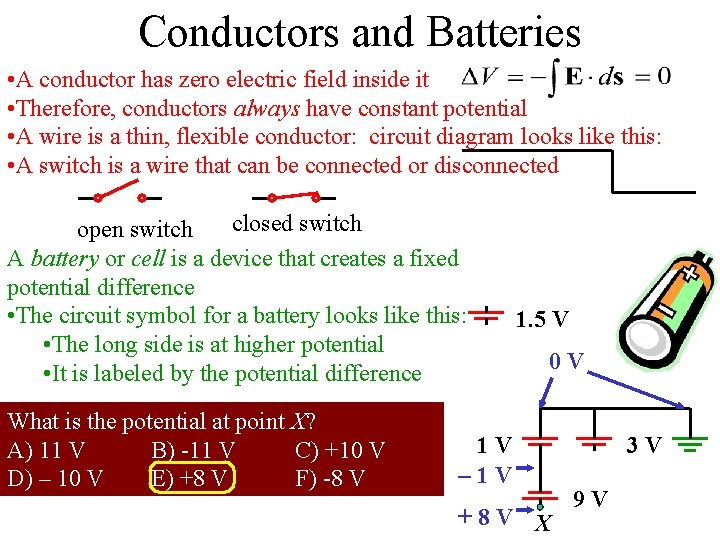Conductors and Batteries • A conductor has zero electric field inside it • Therefore, conductors always have constant potential • A wire is a thin, flexible conductor: circuit diagram looks like this: • A switch is a wire that can be connected or disconnected closed switch open switch A battery or cell is a device that creates a fixed potential difference • The circuit symbol for a battery looks like this: • The long side is at higher potential • It is labeled by the potential difference What is the potential at point X? A) 11 V B) -11 V C) +10 V D) – 10 V E) +8 V F) -8 V 1. 5 V 0 V 1 V – 1 V +8 V X 3 V 9 V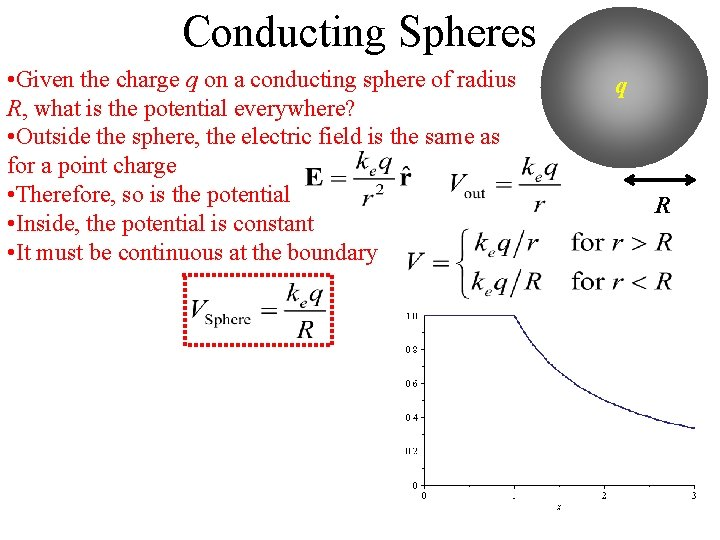Conducting Spheres • Given the charge q on a conducting sphere of radius R, what is the potential everywhere? • Outside the sphere, the electric field is the same as for a point charge • Therefore, so is the potential • Inside, the potential is constant • It must be continuous at the boundary q RSample Problem q 1 Two widely separated conducting spheres, of radii R 1 = 1. 00 cm and R 2 = 2. 00 cm, each have 6. 00 n. C of charge put on them. What is their potential? They are then joined by an electrical wire. How much charge do they each end up with, and what is the final potential? • After connections, their potentials must be equal q 2Warmup 07 25. 19Electric Fields near conductors q 1 q 2 • The potential for the two spheres ended up the same • The electric fields at the surface are not the same • The more curved the surface is, the higher the electric field is there Very strong electric field here • A sharp point can cause charged particles to spontaneously be shed into air, even though we normally think of air as an insulator [ionize air] • Called “Corona discharge”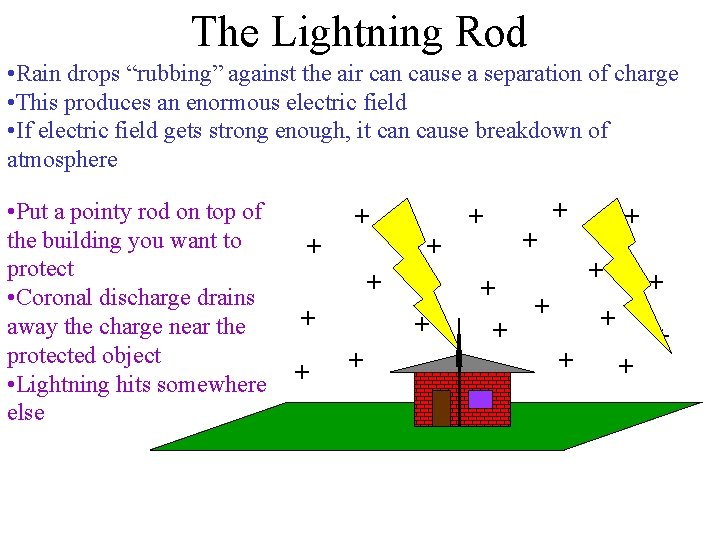The Lightning Rod • Rain drops “rubbing” against the air can cause a separation of charge • This produces an enormous electric field • If electric field gets strong enough, it can cause breakdown of atmosphere • Put a pointy rod on top of the building you want to protect • Coronal discharge drains away the charge near the protected object • Lightning hits somewhere else + + + + + +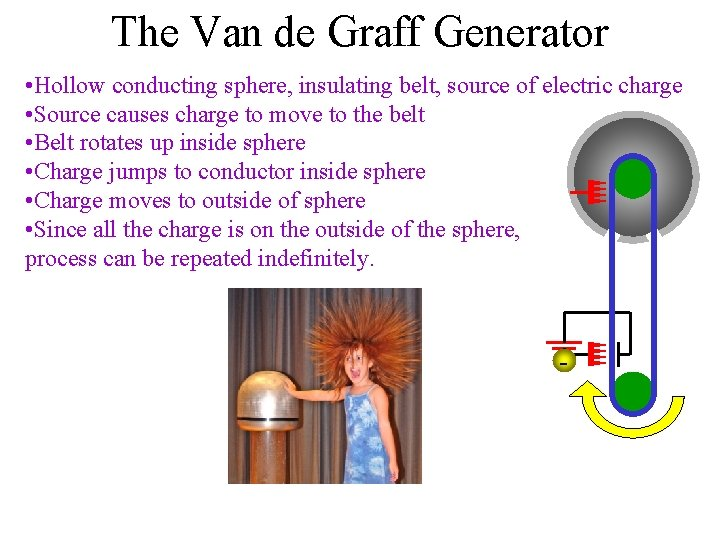The Van de Graff Generator • Hollow conducting sphere, insulating belt, source of electric charge • Source causes charge to move to the belt • Belt rotates up inside sphere • Charge jumps to conductor inside sphere • Charge moves to outside of sphere • Since all the charge is on the outside of the sphere, process can be repeated indefinitely. -